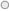## The Salary TheoremDilbert's "Salary Theorem" states that "Engineers and scientists can never earn as much as business executives and sales people."

This theorem can now be supported by a mathematical equation based on the following two postulates:
Postulate 1: Knowledge is Power.
Postulate 2: Time is Money.

As every engineer knows:
Power = Work / Time

And since: Knowledge = Power
And: Time = Money
It is, therefore, true that
Knowledge = Work / Money

Solving this equation for Money, we get:
Money = Work / Knowledge

Thus, as Knowledge approaches zero, Money approaches infinity, regardless of the amount of Work done.

Conclusion: The less you know, the more you make.